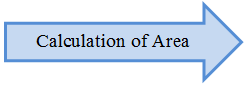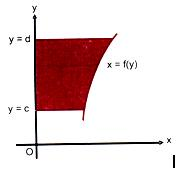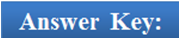×#### Thank you for registering.

One of our academic counsellors will contact you within 1 working day.

Click to Chat

1800-1023-196

+91-120-4616500

CART 0

• 0

MY CART (5)

Use Coupon: CART20 and get 20% off on all online Study Material

ITEM
DETAILS
MRP
DISCOUNT
FINAL PRICE
Total Price: Rs.

There are no items in this cart.
Continue ShoppingArea under Curves – Basic ConceptsThe calculation of area under curves is a very important topic under integral calculus. In such questions it is indispensable to solve a question without the graph. So once you draw a graph, it gives a clear picture of the question and the limits of integration too. Hence, students are advised to focus on their curve tracing skills so as to solve the questions of area under curves easily and quickly.

The working rules to compute area have been discussed in the coming sections. We shall discuss here some of the basic concepts of area under curves:

The procedure to calculate the area under a curve f(x) is given by the formula

A =

The geometrical interpretation of a definite integral is that it gives the area included between the curve y = f(x), the x-axis and the ordinates x = a and x = b.Consider the curve shown in the above figure. We take a strip of width δx and length y from x-axis.

Clearly Area EFGJ < Area FEIG < Area FEIK.

This means yδx < Area FEIG < (y + δy).δx

Also, as δx ≥ 0 the area of lower and upper rectangle tend to be equal. Thus, by sandwich theorem

Area FEIG = ydx or dΔ = ydx.

Hence, the area of the region ABCDA is given by

Some Important Facts

1. Area bounded by the curve y2 = 4ax and x2 = 4by is 16ab/3.

This result can be easily proved by computing the points of intersection of both and then applying the above formula.

2. Area bounded by the parabola y2 = 4ax and y = mx is equal to 8a2/3m3.3. Area enclosed between the curve y2 = 4ax and its double ordinate at x = a is 8a2/3:4. The area of the whole ellipse is equal to πab.5. Let f(x) be a continuous function in (a, b). Then the area bounded by the curve y = f(x), the x-axis and the lines x = a and x = b is given by the formulae

provided f(x) > 0 (or f(x) < 0)) ∀ x ∈ (a, b).6. The area bounded by x = f(y), the y-axis and the lines y = c and y = d is given by

provided f(y) > 0 (or f(y) < 0)) ∀ y ∈ (a, b).7. The area enclosed by a curve remains invariant if the coordinate axis are shifted and hence shifting of origin can be utilized in attempting such questions as it simplifies the computation of area.

8. In order to draw the curve, some standard steps to be followed are:

(a) Check the symmetry:

If when y is changed to (-y), the curve remains unchanged, then the curve is said to be symmetrical about the x-axis.

If when x is changed to (-x), the curve remains unchanged, then the curve is said to be symmetrical about the x-axis.

(iii) Symmetry about both the axis:

If the curve remains unchanged when x is changed to (-x) and when y is changed to (-y) then the curve is said to be symmetrical about both the axis.

(iv) Symmetry about the line y = x:

If the curve remains unchanged on interchanging x and y, then the curve is said to be symmetrical about the line y = x.

(b) Figure out the points where the curve intersects the x and the y-axis.

(c) Compute dy/dx to identify the stationary points. If possible, try to search for the intervals where f(x) is increasing or decreasing.

(d) Try to identify y when x → ∞ or -∞.

The above cases generally cover most of the questions of area. It is advised to shade the area in the graph as done above so that it becomes simple to get the limits and the chances of committing errors are also minimized.

Watch this Video for more referenceQ1. A curve is said to be symmetrical about the x-axis if

(a) interchanging x by (-x) gives the same curve

(b) interchanging y by (-y) gives the same curve

(c) it has even powers of x.

(d) None of these

Q2. Area bounded by the parabola y2 = 4ax and y = mx is equal to

(a) 8a2/3m3

(b) 8m2/3a3

(c) 8a/3m3

(d) 8a3/ 3m2

Q3. Area of an ellipse is

(a) πab

(b) πab/6

(c) πb/6a

(d) 6π/ba

Q4. In order to compute the stationary points, one must find out

(a) d2y/dx2

(b) points at which function is zero.

(c) dy/dx

(d) None of these

Q5: On shifting the coordinate axis, the area of the curve

(a) increases

(b) decreases

(c) can’t say

(d) remains unchanged.1(b), 2(a), 3(a), 4(c), 5(d).

We now discuss some of the illustrations to get an idea about the kind of questions asked in JEE:

Illustration 1:

The triangle formed by the tangent to the curve f(x) = x2 + bx – b at the point (1,1) and the coordinate axis lies in the first quadrant. If its area is 2, then what is the value of b?

Solution:

Let y = f(x) = x2 + bx – b

The equation of the tangent at P (1, 1) to the curve 2y = 2x2 + 2bx – 2b is

y +1 = 2x.1 + b (x+1) - 2b

so, y = (2 + b)x – (1 + b)

It meets the coordinate axis at xA = (1 + b)/(2 + b) and yB = – (1 + b)

Hence, the area of ?OAB = 1/2 OA x OB = -1/2 (1 + b)2 / (2+b) = 2

This means (1 + b)2 + 4(2 + b) = 0.

b2 + 6b + 9 = 0

(b + 3)2 = 0

So, b = -3. Hence, this is the required value of b.

___________________________________________________________________________________

Illustration 2:

Let An be the area bounded by the curve y = (tan x)n and the lines x = 0, y = 0 and x = π/4. Prove that for n > 2, An + An-2 = 1/(n-1) and deduce that 1/ (2n + 2) < An < 1/(2n-2) ∀n.

Solution:

Required area bounded by the curve y = (tan x)n between x = 0, y = 0 and x = π/4 is

An = ∫ tannx dx, where the integral runs from 0 to π/4.

An = ∫ tann-2x (sec2x -1) dx, integral runs from 0 to π/4.

An = 1/(n-1) – 0 – An-2

An + An-2 = 1/(n-1)  …… (1)

In (0, π/4), tannx < tann-2x and tann+2x < tannx

Hence, ∫ tannx dx < ∫ tann-2x dx, here integral runs from 0 to π/4

And ∫ tann+2x dx < ∫ tannx dx, here integral runs from 0 to π/4

So, An < An-2 and An+2 < An

By adding An, 2An < An + An-2

And An + An+2 < 2An

2An < 1/(n-2) and 1/(n+1) < 2 An

Therefore, 1/(n+1) < 2An < 1/(n-2)

Hence, 1/(2n+2) < An < 1/(2n-2) ∀n.

___________________________________________________________________________________

Illustration 3:

Find the area enclosed within the curve |x| + |y| =1.

Solution:

The given curve is |x| + |y| =1.

This curve can be broken in the form of following four lines

x+y = 1

-x+y = 1

-x-y = 1

x-y = 1

Plotting these four lines on a graph, it can be seen that they form a square with vertices at points (0, 1), (1, 0), (-1, 0) and (0,-1).

Hence, the required area = Area of the square = (√2)2 = 2 sq. unit.

Related Resources

You may wish to refer definite integral.

For getting an idea of the type of questions asked, refer the previous year papers.

To read more, Buy study materials of Application of Integration comprising study notes, revision notes, video lectures, previous year solved questions etc. Also browse for more study materials on Mathematics here.### Course Features

• 731 Video Lectures
• Revision Notes
• Previous Year Papers
• Mind Map
• Study Planner
• NCERT Solutions
• Discussion Forum
• Test paper with Video Solution